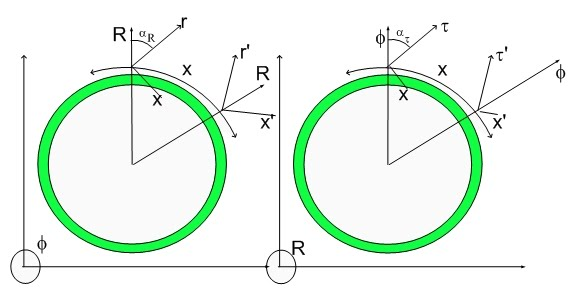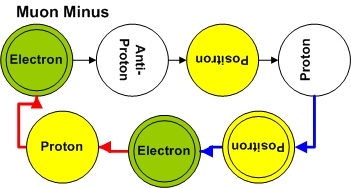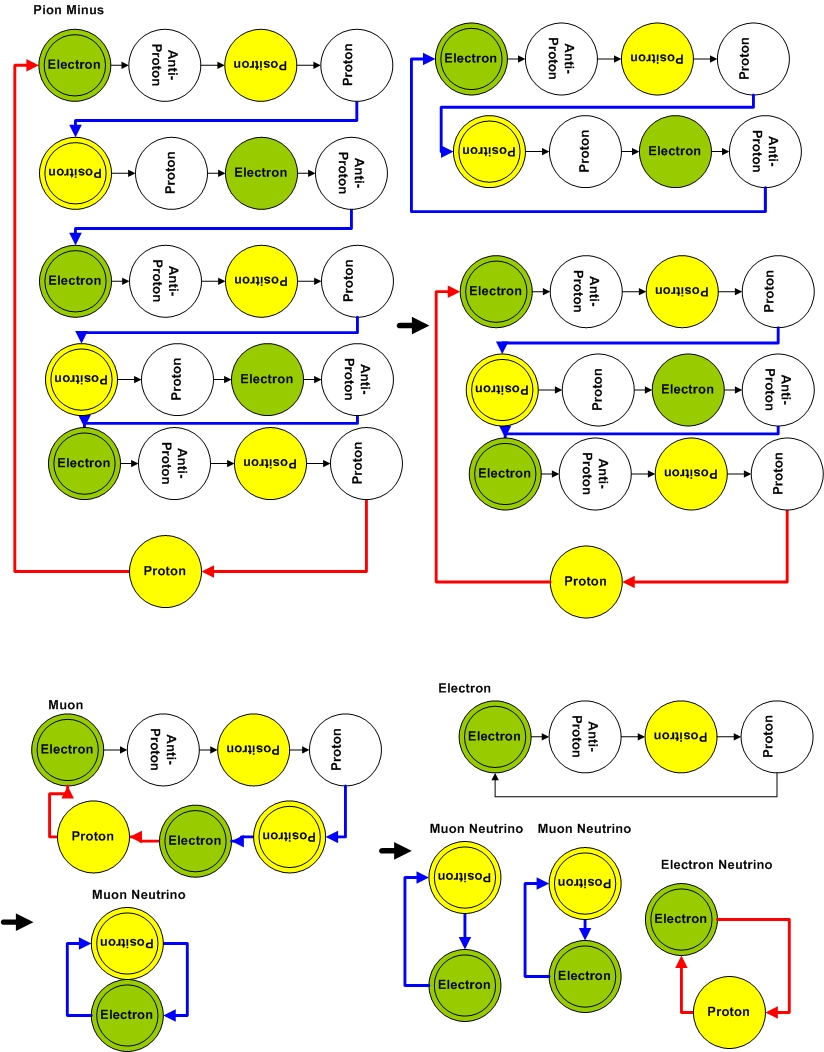# Pion Decay along Path TwoPion Decay along Path Two

The prior assignment for Muon Minus in my theory was given by:Of course, this assignment was designed to be consistent with the decay pathway leading to an electron, an electron neutrino and a muon neutrino.

This would be consistent with a different initial Pion Glutonic State, that is, a Pion that swallow an extra electron-positron pair...:)Along this pathway, a Pion in the First Glutonic State decays into an Muon Minus and an Muon Neutrino. The Muon Minus then decays into an electron, an muon neutrino and an electron neutrino. This means that along this pathway, the Pion decays into an electron, two muon neutrinos and an electron neutrino.

Where here one would start with a pentameric pion minus (as opposed to a trimeric moiety) resulting in the expected neutrino ratio. This might be a better assignment for the pion state associated with the muon pathway. Current experiments are not sensitive enough to distinguish a trimeric from a pentameric pion. Assignments are tricky if one considers the lack of precision on the determination of the initial state of any decay reaction (e.g. Pion decay). Since the only discrepancy is the Pion Minus (trimeric) decaying into a muon Minus, I will consider that reaction to be starting from a Pion Minus* state (Pentameric Pion Minus).

Experiments that would probe the deformation susceptibility of the Fabric of Space are eons in the future - if not longer...:) They would be happening now if there wasn't such a horrendous censorship in Science.

Of course, those experiments would use the Coherent Fusion scheme I proposed...:)

As soon as I have some time, I will present how to calculate the masses of everything and how to model Fabric of Space Deformation Susceptibility...:)

Cheers,

MP

Currently unrated

### New Comment

required

required (not published)

optional

required